Keyah Math Module 9, Level 3

Mathematical Content: Basic algebra, formulae for gravitational attraction

#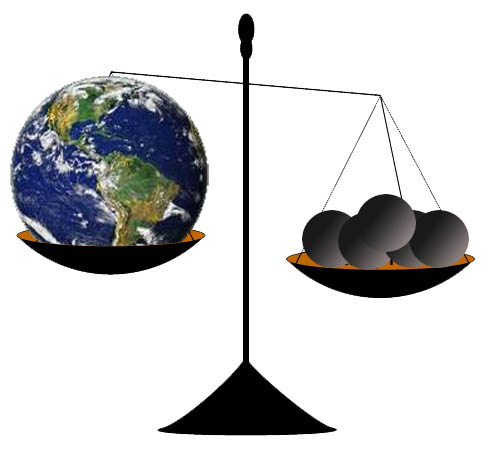Determining the Mass and Density of the Earth:

The goal of this study is to learn how the famous physicist, Sir Isaac Newton, computed the mass of the Earth, and then use this to compute its density.

Basic Concepts:

* Any object in the Universe attracts any other object.

* The force that moves objects toward each other is called gravity.

* The mass of an object is a fundamental property of the object with measured in kilograms (kg).

* Even though we often use weight and mass interchangeably in everyday language, the weight of an object depends on the force of gravity. If you went to the moon, your mass would not change but your weight would be much less. The weight of an object is the force of gravity on the object; this can be described by the equation

w = mg where m is the mass of the object and g is the acceleration due to gravity.

* The density of an object is the mass per unit volume.

In the year 1680, Sir Isaac Newton discovered the famous equation known as the Law of Gravitational Attraction on two objects. You will use this result, together with another result also due to Newton, to compute the mass of the Earth.

 Notation: Newton ’s Law of Gravitational Attraction,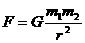, describes the force with which two objects attract each other. G is called the universal gravitational constant and its value is known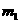and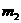denote the masses of the two objects r is the distance between the objects. For an object with mass m on the surface of the earth, this equation becomes Equation 1: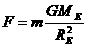where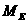is the mass of the earth and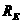is the radius of the earth. Newton’s Second Law of Motion, F = mass x acceleration describes the relationship between force, mass and acceleration. On the surface of the earth the acceleration of gravity is about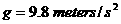and we have Equation 2: F = mg. Since equations 1 and 2  describes the same force, we have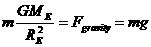,  which simplifies to our basic equation Basic Equation: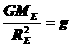.

Thought questions

• If the distance between two objects increases does the force of attraction increase or decrease?

• Which object will have greater acceleration due to gravity, one of very large mass or one of very small mass?

You will use the basic equationto estimate the mass, volume and density of the earth.

 Information: The average radius of the Earth is 6.38 x 106 meters The universal gravitational constant G is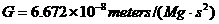where Mg is megagrams (1 Mg = 1000 kilograms) and s is seconds. The acceleration of gravity at the surface of the Earth is aboutThe volume of a sphere of radius r is given by the formula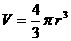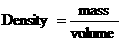The answers to the following questions will use Newton’s methods to determine the mass and density of the Earth.

 Question 1: What is the mass of the Earth? To answer this question, follow these steps: Substitute the values given above in the equation. Solve the equation for ME, the mass of the Earth.

 Question 2 What is the volume of the Earth? To answer this question, assume the Earth is a sphere with radius RE. Use the appropriate information given above.

 Question 3 What is the density of the Earth? Use your answers from the first two questions to answer this one.

Here are some follow-up questions to think about:

 How do you think Newton discovered his Law of Gravitational Attraction? Do you think this is an accurate method for computing the density of the Earth? Do you know of modern, more high-tech techniques that might give a more accurate result? How do you think geologists might measure the density of rocks? Why do you think it's important to know the density of the Earth, or rocks that are part of the Earth? What do you think the Earth is composed of to give it the density that you calculated?

This material is based upon work supported by the National Science Foundation under Grant GEO-0355224. Any opinions, findings, and conclusions or recommendations expressed in this material are those of the authors and do not necessarily reflect the views of the National Science Foundation.